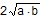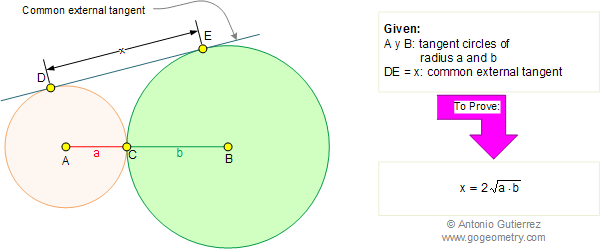Problem 277: Tangent Circles, Common External Tangent Level: High School, SAT Prep, College geometry. In the figure below, the circles of centers A and B with radius a and b, respectively, are tangent externally at point C. If DE is the common external tangent, prove that DE =.Recent Additions